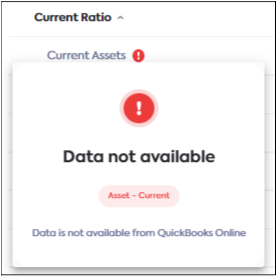# Indicators

### 🚧Indicators - Beta testing

Note that Indicators is still in testing mode across all integrations and hasn't been fully released yet.

If you're interested in participating in the beta testing, contact your sales representative.

This feature provides a set of pre-calculated ratios and metrics used to assess company financial performance. It leverages the Categories feature, which standardizes bespoke data across SMEs. After the data is standardized, meaningful insights and measurements are produced for financial performance.

Currently, to measure company performance, clients need to map each of their customer’s bespoke financial statements to a single standardized chart of accounts - this is what our Categories feature does. After financials are fully standardized, clients need to perform complex calculations in order to produce financial ratios and metrics - this is what our Indicators feature does. It saves the client considerable time and effort.

With this feature, you will decrease the time to onboard customers, underwrite customers faster, easily monitor the ongoing health of your customers, and provide improved financial reporting, planning and analysis. You will have more confidence in your decisions knowing they are based on the latest, most accurate data available.

# What ratios and metrics are available?

The following table lists which ratios and metrics are calculated, their formulas and how they translate to Codat data points:

Ratio / Metric

Formula

How that's translated to Codat data points

Gross profit margin

gross profit / net sales

Gross profit = a-b

• a. sum[Income.Operating]
• b. sum[Expense.CostOfSales]
Net Sales = a
• a. sum[Income.Operating]

EBITDA

net income + interest + tax + depreciation + amortization

Net Income = a - b

• a. sum [Income]
• b. sum [Expense]
Interest = a + b
• a. sum[Expense.Operating.Interest]
• b. sum[Expense.NonOperating.Interest]
Tax = a
• a. sum[Expense.NonOperating.Tax]
Depreciation = a
• a. sum[Expense.Operating.DepreciationDepletion]
Amortization = a
• a. sum[Expense.Operating.Amortization]

Debt service coverage ratio

net operating income/total debt service

Where,
total debt service = Loan interest + principal

Net Operating Income = a - (b + c)

• a. sum[Income.Operating]
• b. sum[Expense.CostOfSales]
• c. sum[Expense.Operating]
Total Debt Service = sum(a : n)
• a. sum[Expense.NonOperating.Interest]
• b. sum[Expense.Operating.Interest]
• c. sum[Expense.Operating.EquipmentRentLease]
• d. sum[Expense.Operating.BuildingRentLease]
• e. sum[Liability.Current.NotesPayable]
• f. sum[Liability.Current.LoansPayable]
• g. sum[Liability.Current.InterestPayable]
• h. sum[Liability.Current.FinanceLeaseObligations]
• i. sum[Liability.Current.CapitalLeaseObligations]
• j. sum[Liability.Current.OtherShortTermDebt]
• k. sum[Liability.Current.OtherLongTermDebt]
• l. sum[Liability.Current.CreditCards]
• m. sum[Liability.Current.ConvertibleDebt]

Current ratio

current assets / current liabilities

Current Assets = a

• a. sum[Asset.Current]
Current Liabilites = a
• a. sum[Liability.Current]

Quick ratio

(current assets - inventory – prepaid expenses) / current liabilities

Current Assets = a

• a. sum[Asset.Current]
Inventory = sum(a : e)
• a. sum[Asset.Current.InventoryMerchandise]
• b. sum[Asset.Current.InventoryFinishedGoods]
• c. sum[Asset.Current.OtherInventory]
• d. sum[Asset.Current.InventoryRawMaterials]
• e. sum[Asset.Current.InventoryWorkInProcess]
Prepaid Expenses = a
• a. sum[Asset.Current.PrepaidExpenses]
Current Liabilities = a
• a. sum[Liability.Current]

Free cash flow

net income + Depreciation and amortization (non-cash expense) - Working Capital Variation - Purchases of PP&E (CAPEX)

Where,
Working Capital Variation = Stock + Account Receivable (trade and others) – Account Payables (trade and others)

Net Income = a - b

• a. sum [Income]
• b. sum [Expense]
Non-cash expense = sum(a:d)
• a. sum[Expense.Operating.DepreciationDepletion]
• b. sum[Expense.Operating.Amortization]
• c. sum[Expense.Operating.RevaluationImpairment]
• d. sum[Expense.Operating.EmployeeStockCompensation]
Working Capital variation = ((sum(a:e) + k ) - m) - ((sum(f:j) + l) - n)
• a. sum[Asset.Current.InventoryMerchandise]
• b. sum[Asset.Current.InventoryFinishedGoods]
• c. sum[Asset.Current.OtherInventory]
• d. sum[Asset.Current.InventoryRawMaterials]
• e. sum[Asset.Current.InventoryWorkInProcess]
• f. PreviousYr'sum[Asset.Current.InventoryMerchandise]
• g. PreviousYr'sum[Asset.Current.InventoryFinishedGoods]
• h. PreviousYr'sum[Asset.Current.OtherInventory]
• i. PreviousYr'sum[Asset.Current.InventoryRawMaterials]
• j. PreviousYr'sum[Asset.Current.InventoryWorkInProcess]
• k. sum[Asset.Current.AccountsReceivable]
• l. PreviousYr'sum[Asset.Current.AccountsReceivable]
• m. sum[Liability.Current.AccountsPayable]
• n. PreviousYr'sum[Liability.Current.AccountsPayable]
Purchases of PP&E = sum(a:j) - sum(k:t)
• a. sum[Asset.NonCurrent.FixturesFittings]
• b. sum[Asset.NonCurrent.EquipmentMachinery]
• c. sum[Asset.NonCurrent.LandBuildings]
• d. sum[Asset.NonCurrent.LandBuildingsImprovements]
• e. sum[Asset.NonCurrent.OtherTangibleAssets]
• f. sum[Asset.NonCurrent.CapitalLeases]
• g. sum[Asset.NonCurrent.AssetsUnderConstruction]
• h. sum[Asset.NonCurrent.Goodwill]
• i. sum[Asset.NonCurrent.IntangibleAssets]
• j. sum[Asset.NonCurrent.AcquisitionsInProgress]
• k. PreviousYr'sum[Asset.NonCurrent.FixturesFittings]
• l. PreviousYr'sum[Asset.NonCurrent.EquipmentMachinery]
• m. PreviousYr'sum[Asset.NonCurrent.LandBuildings]
• n. PreviousYr'sum[Asset.NonCurrent.LandBuildingsImprovements]
• o. PreviousYr'sum[Asset.NonCurrent.OtherTangibleAssets]
• p. PreviousYr'sum[Asset.NonCurrent.CapitalLeases]
• q. PreviousYr'sum[Asset.NonCurrent.AssetsUnderConstruction]
• r. PreviousYr'sum[Asset.NonCurrent.Goodwill]
• s. PreviousYr'sum[Asset.NonCurrent.IntangibleAssets]
• t. PreviousYr'sum[Asset.NonCurrent.AcquisitionsInProgress]

Working capital

current assets – current liabilities

Current Assets = a

• a. sum[Asset.Current]
Current Liabilites = a
• a. sum[Liability.Current]

Fixed-charge coverage ratio

(EBIT + Fixed charge before tax) / (Fixed charge before tax + Interest)

EBIT = (a - b - c) + d

• a. sum[Income.Operating]
• b. sum[Expense.CostOfSales]
• c. sum[Expense.Operating]
• d. sum[Expense.Operating.Interest]
Fixed charge before tax = sum(a:p)
• b. sum[Expense.Operating.SubscriptionFees]
• c. sum[Expense.Operating.OtherEmployeeBenefits]
• d. sum[Expense.Operating.EmployeeWages]
• e. sum[Expense.Operating.BuildingRentLease]
• f. sum[Expense.Operating.EquipmentRentLease]
• g. sum[Expense.Operating.BankCharges]
• h. sum[Expense.Operating.ManagementFees]
• i. sum[Expense.Operating.ServiceChargeGroundRent]
• j. sum[Expense.Operating.DirectorsCompensation]
• k. sum[Expense.Operating.EmployeeTax]
• l. sum[Expense.Operating.Pension]
• m. sum[Expense.Operating.DepreciationDepletion]
• n. sum[Expense.Operating.Amortization]
• o. sum[Expense.Operating.EmployeeStockCompensation]
• p. sum[Expense.Operating.RevaluationImpairment]
Interest = a
• a. sum[Expense.Operating.Interest]

# How to access Indicators

You can access Indicators on the Codat Portal. Make sure you have Accounts, Profit and Loss, and Balance Sheet enabled to sync.

2. Go to the Companies page.
3. Click the company whose ratios and metrics you want to calculate.
4. Click the Visualize button.
Skip the Companies House popup.
5. Click the Profit and Loss tab or the Balance Sheet tab.
6. Click the Indicators tab which is located below the graph and to the left. The following table of ratios and metrics displays below the graph: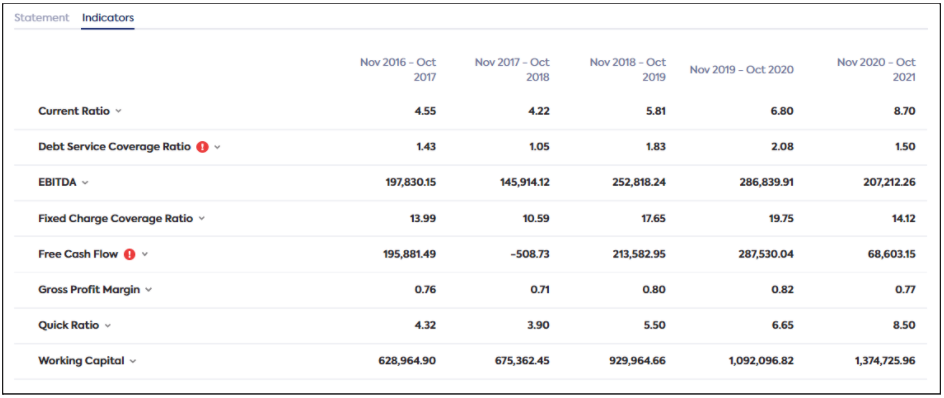1. Specify your filters: Latest Month, Period Length and Periods to Compare, to automatically calculate the ratios and metrics for your selection.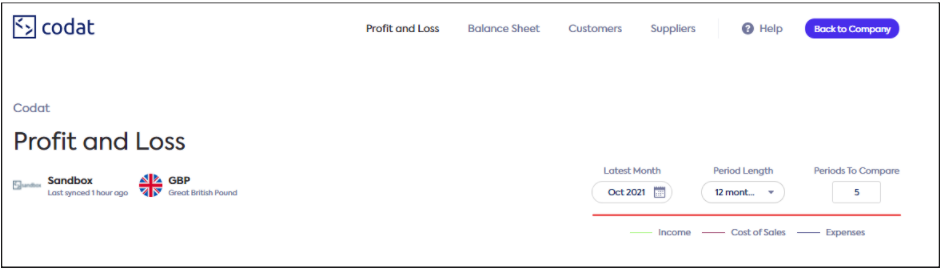# Resolve errors

Errors are flagged with a red and white exclamation mark as shown in the image below. The system generates the following errors:

• Accounts are missing categories
• Data not available

### Accounts are missing categories

This error occurs when an account is either uncategorized or is partially categorized.

When a company is first linked, Codat automatically categorizes accounts and attempts to calculate the ratios and metrics. If an account could not be auto-categorized, it will show an error message. Clicking the message and following the instructions will guide you through a short process to manually apply a category to the account. Once completed, the ratios and metrics are recalculated automatically. The steps for doing this are shown below.

1. Click the exclamation mark to view the error message.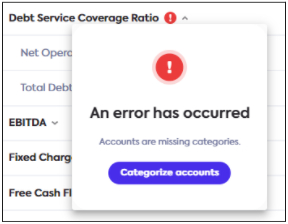1. Click the Categorize accounts button. The screen below is displayed.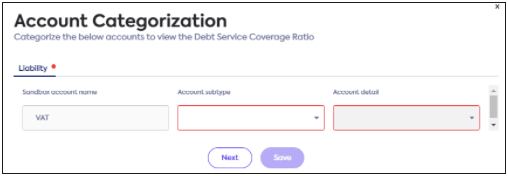In this example, it shows that the VAT account has not been categorized.

1. Select an Account subtype, Account detail and click Save.
The ratios and metrics will automatically recalculate.

### Data not available

If a company does not have accounts that a ratio or metric usually requires, it is treated as zero and flagged with a red and white exclamation mark. This warning is for information purposes only, no user action is required. For example, the current ratio is computed using formula current assets/current liabilities. If a business does not have any accounts which are considered current assets, that line item will show the warning icon.